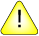This version of this document is no longer maintained. For the latest documentation, see http://www.qnx.com/developers/docs.

# finite(), finitef()

Determine if a number is finite

## Synopsis:

```#include <math.h>

int finite ( double x );

int finitef ( float x);```

## Arguments:

x
The number you want to test.

## Library:

libm

Use the -l m option to qcc to link against this library.

## Description:

The finite() and finitef() functions determine if x is finite.

## Returns:

True (1)
The value of x is finite.
False (=/= 1)
The value of x is infinity or NAN.

## Examples:

```#include <stdio.h>
#include <errno.h>
#include <inttypes.h>
#include <math.h>
#include <fpstatus.h>

int main(int argc, char** argv)
{
double a, b, c, d;

a = 2;
b = -0.5;
c = NAN;
d = 1.0/0.0;
printf("%f is %s \n", a, (finite(a)) ? "finite" : "not-finite");
printf("%f is %s \n", b, (finite(b)) ? "finite" : "not-finite");
printf("%f is %s \n", c, (finite(c)) ? "finite" : "not-finite");
printf("%f is %s \n", d, (finite(d)) ? "finite" : "not-finite");

return(0);
}```

produces the output:

```2.000000 is finite
-0.500000 is finite
NAN is not-finite
Inf is not-finite```

## Classification:

Unix

Safety:
Cancellation point No
Interrupt handler No
Signal handler No